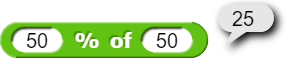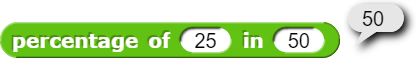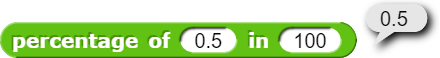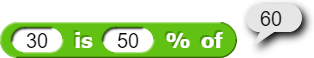# Get the percentage of a number in a bigger number

I figured out how to calculate the percentage of a number in another number, and accidentally found out how to get a number percentage of another number... that was terrible. Here's the blocks and I think you can figure out what they do.Here's the project

I hate myself for taking over one week to figure out how to do this, it's super simple.

I think it'd be better if the
[scratchblocks]
(percentage of (25) in (50)::operators)
[/scratchblocks]
block returned 0.5 instead of 50. Percentages are really numbers usually in the range of 0 to 1, so I think it'd be better to return that. It will also make it easier to do math with a percentage representing a number between 0 and 1 instead of a number between 0 and 100.

um...

In mathematics, a percentage is a number or ratio that represents a fraction of 100. It is often denoted by the symbol "%" or simply as "percent" or "pct." For example, 35% is equivalent to the decimal 0.35, or the fraction. 35.

Uh... yeah? Is this a counterpoint or something? You're confirming what I said.

sorry, I didn't actually read the end of the quote, when i posted it, but what happens when you dowould that just report 0.005? I also want it to report the whole number between 0 and 100. If you really want it to report a decimal between 0 and 1, then you can just do

25 is not 0.5% of 50.

100% = 1

Don't change the inputs, only change the output. The inputs aren't percents.

But 25 is 50% of 50. And 50% = 0.5.

Sorry it took forever to reply, the reply cooldown prevented me from replying to your post. I wanted to make it so it looked like I was replying to you on the top right corner.

Umm...It appears very clearly to me that the first input of this block is a percent (there's even a percent sign). If it wasn't a percent, then the result would be the square of 50 in this example.

Sorry, I meant only for the percentage of block, not the % of block. The first input in the % of block is clearly a percent. Ugh, darn reply cooldown...